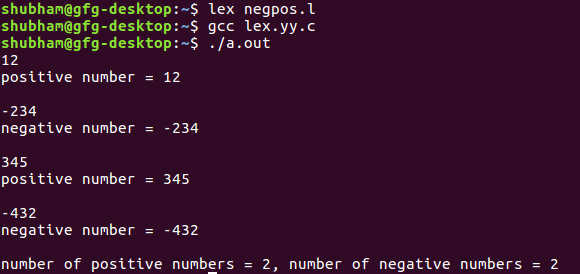# Lex Program to Identify and Count Positive and Negative Numbers

Given some numbers, task is to identify the positive and negative numbers and print the count of negative and positive numbers.

Prerequisite: Flex (Fast lexical Analyzer Generator)

Examples:

```Input : -52
Output :
negative number=-52
number of positive numbers = 0
number of negative numbers = 1

Input : 63
Output :
positive number = 63
number of positive numbers = 0
number of negative numbers = 1
```

Below is the implementation program:

 `/* Lex program to Identify and Count  ` `Positive and Negative Numbers */`  `%{ ` `int` `positive_no = 0, negative_no = 0; ` `%} ` `  `  `/* Rules for identifying and counting  ` `positive and negative numbers*/` `%% ` `^[-][0-9]+ {negative_no++;  ` `            ``printf``(``"negative number = %s\n"``, ` `                  ``yytext);}  ``// negative number ` ` `  `[0-9]+ {positive_no++; ` `        ``printf``(``"positive number = %s\n"``,  ` `                 ``yytext);} ``// positive number      ` `%%  ` ` `  `/*** use code section ***/` ` `  `int` `yywrap(){} ` `int` `main()                                               ` `{ ` `  `  `yylex();  ` `printf` `(``"number of positive numbers = %d,"` `        ``"number of negative numbers = %d\n"``, ` `                ``positive_no, negative_no); ` ` `  `return` `0;  ` `} `

Output:My Personal Notes arrow_drop_upCheck out this Author's contributed articles.

If you like GeeksforGeeks and would like to contribute, you can also write an article using contribute.geeksforgeeks.org or mail your article to contribute@geeksforgeeks.org. See your article appearing on the GeeksforGeeks main page and help other Geeks.

Please Improve this article if you find anything incorrect by clicking on the "Improve Article" button below.

Article Tags :

Be the First to upvote.

Please write to us at contribute@geeksforgeeks.org to report any issue with the above content.